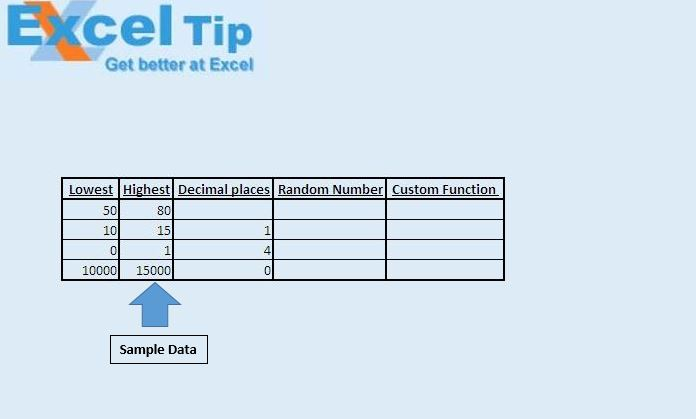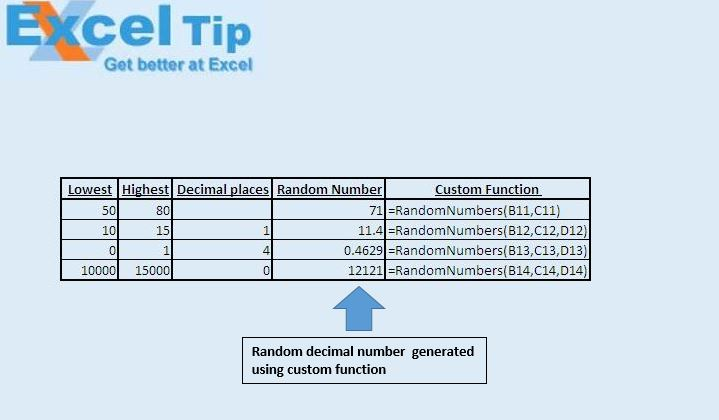# An Improved Custom Random Numbers Function

In this article, we will create a custom function to generate random decimal numbers between the defined ranges.

Raw data for this example consists of lowest value and highest value of the range and number of decimal places required in decimal numbers.We have created a custom function “RandomNumbers” to generate random decimal numbers between the defined lowest and highest numbers. This function takes lowest value, highest value and number of decimal places required as input and returns random decimal numbers as output.Code explanation

Randomize function

Randomize function is used to initialize the RnD function of the random-number generator and assign it a new seed value.

RnD function

RnD function returns a random number of single data type.

Round function

Round function is used to limit the number of decimal places.

Syntax of Round function

Round(value,Number_of_decimal_places)

```
Option Explicit
Function RandomNumbers(Lowest As Double, Highest As Double, _
Optional Decimals As Integer = 0)

Randomize

RandomNumbers = Round((Highest - Lowest) * Rnd + Lowest, Decimals)

End Function

```